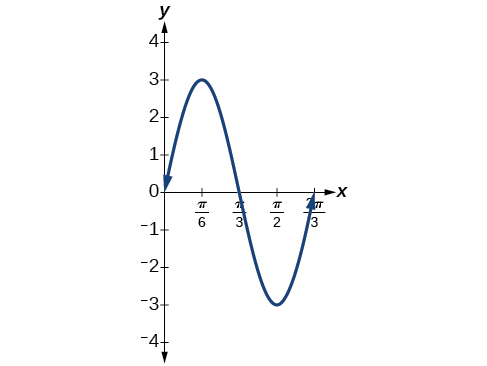# 7.6 Modeling with trigonometric equations  (Page 2/14)

 Page 2 / 14

## Finding the amplitude and period of a function

Find the amplitude and period of the following functions and graph one cycle.

1. $y=2\text{\hspace{0.17em}}\mathrm{sin}\left(\frac{1}{4}x\right)$
2. $y=-3\text{\hspace{0.17em}}\mathrm{sin}\left(2x+\frac{\pi }{2}\right)$
3. $y=\mathrm{cos}\text{\hspace{0.17em}}x+3$

We will solve these problems according to the models.

1. involves sine, so we use the form
$y=A\text{\hspace{0.17em}}\mathrm{sin}\left(Bt+C\right)+D$

We know that is the amplitude, so the amplitude is 2. Period is so the period is

See the graph in [link] .

2. involves sine, so we use the form
$y=A\text{\hspace{0.17em}}\mathrm{sin}\left(Bt-C\right)+D$

Amplitude is so the amplitude is Since is negative, the graph is reflected over the x -axis. Period is so the period is

$\frac{2\pi }{B}=\frac{2\pi }{2}=\pi$

The graph is shifted to the left by units. See [link] .

3. involves cosine, so we use the form
$y=A\text{\hspace{0.17em}}\mathrm{cos}\left(Bt±C\right)+D$

Amplitude is so the amplitude is 1. The period is See [link] . This is the standard cosine function shifted up three units.

What are the amplitude and period of the function

The amplitude is and the period is

## Finding equations and graphing sinusoidal functions

One method of graphing sinusoidal functions is to find five key points. These points will correspond to intervals of equal length representing of the period. The key points will indicate the location of maximum and minimum values. If there is no vertical shift, they will also indicate x -intercepts. For example, suppose we want to graph the function We know that the period is $\text{\hspace{0.17em}}2\pi ,$ so we find the interval between key points as follows.

$\frac{2\pi }{4}=\frac{\pi }{2}$

Starting with we calculate the first y- value, add the length of the interval to 0, and calculate the second y -value. We then add repeatedly until the five key points are determined. The last value should equal the first value, as the calculations cover one full period. Making a table similar to [link] , we can see these key points clearly on the graph shown in [link] .

 $\theta$ $0$ $\frac{\pi }{2}$ $\pi$ $\frac{3\pi }{2}$ $2\pi$ $y=\mathrm{cos}\text{\hspace{0.17em}}\theta$ $1$ $0$ $-1$ $0$ $1$

## Graphing sinusoidal functions using key points

Graph the function using amplitude, period, and key points.

The amplitude is The period is (Recall that we sometimes refer to as One cycle of the graph can be drawn over the interval To find the key points, we divide the period by 4. Make a table similar to [link] , starting with and then adding successively to and calculate See the graph in [link] .

 $x$ $0$ $\frac{1}{2}$ $1$ $\frac{3}{2}$ $2$ $y=-4\text{\hspace{0.17em}}\mathrm{cos}\left(\pi x\right)$ $-4$ $0$ $4$ $0$ $-4$

Graph the function using the amplitude, period, and five key points.

x $3\mathrm{sin}\left(3x\right)$
0 0
$\frac{\pi }{6}$ 3
$\frac{\pi }{3}$ 0
$\frac{\pi }{2}$ $-3$
$\frac{2\pi }{3}$ 0## Modeling periodic behavior

We will now apply these ideas to problems involving periodic behavior.

## Modeling an equation and sketching a sinusoidal graph to fit criteria

The average monthly temperatures for a small town in Oregon are given in [link] . Find a sinusoidal function of the form $\text{\hspace{0.17em}}y=A\text{\hspace{0.17em}}\mathrm{sin}\left(Bt-C\right)+D\text{\hspace{0.17em}}$ that fits the data (round to the nearest tenth) and sketch the graph.

Month Temperature, ${}^{\text{o}}\text{F}$
January 42.5
February 44.5
March 48.5
April 52.5
May 58
June 63
July 68.5
August 69
September 64.5
October 55.5
November 46.5
December 43.5

Recall that amplitude is found using the formula

Thus, the amplitude is

The data covers a period of 12 months, so $\text{\hspace{0.17em}}\frac{2\pi }{B}=12\text{\hspace{0.17em}}$ which gives $\text{\hspace{0.17em}}B=\frac{2\pi }{12}=\frac{\pi }{6}.$

The vertical shift is found using the following equation.

Thus, the vertical shift is

So far, we have the equation $\text{\hspace{0.17em}}y=13.3\text{\hspace{0.17em}}\mathrm{sin}\left(\frac{\pi }{6}x-C\right)+55.8.$

To find the horizontal shift, we input the $\text{\hspace{0.17em}}x\text{\hspace{0.17em}}$ and $\text{\hspace{0.17em}}y\text{\hspace{0.17em}}$ values for the first month and solve for $\text{\hspace{0.17em}}C.$

We have the equation $\text{\hspace{0.17em}}y=13.3\text{\hspace{0.17em}}\mathrm{sin}\left(\frac{\pi }{6}x-\frac{2\pi }{3}\right)+55.8.\text{\hspace{0.17em}}$ See the graph in [link] .

a colony of bacteria is growing exponentially doubling in size every 100 minutes. how much minutes will it take for the colony of bacteria to triple in size
I got 300 minutes. is it right?
Patience
no. should be about 150 minutes.
Jason
It should be 158.5 minutes.
Mr
ok, thanks
Patience
what is the importance knowing the graph of circular functions?
can get some help basic precalculus
What do you need help with?
Andrew
how to convert general to standard form with not perfect trinomial
can get some help inverse function
ismail
Rectangle coordinate
how to find for x
it depends on the equation
Robert
whats a domain
The domain of a function is the set of all input on which the function is defined. For example all real numbers are the Domain of any Polynomial function.
Spiro
foci (–7,–17) and (–7,17), the absolute value of the differenceof the distances of any point from the foci is 24.
difference between calculus and pre calculus?
give me an example of a problem so that I can practice answering
x³+y³+z³=42
Robert
dont forget the cube in each variable ;)
Robert
of she solves that, well ... then she has a lot of computational force under her command ....
Walter
what is a function?
I want to learn about the law of exponent
explain this
what is functions?
A mathematical relation such that every input has only one out.
Spiro
yes..it is a relationo of orders pairs of sets one or more input that leads to a exactly one output.
Mubita
Is a rule that assigns to each element X in a set A exactly one element, called F(x), in a set B.
RichieRich
If the plane intersects the cone (either above or below) horizontally, what figure will be created?

#### Get Jobilize Job Search Mobile App in your pocket Now!ByByBy Robert MurphyBy OpenStaxBy OpenStaxBy Tony PizurBy Laurence BailenBy Cath YuBy Joanna SmithbackBy Brooke DelaneyBy Qqq QqqBy OpenStax